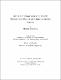## 2D to 3D conversion with direct geometrical search and approximation spaces##### Date
2007-09-14T17:24:19Z
##### Authors
Borkowski, Maciej
##### Abstract
This dissertation describes the design and implementation of a system that has been designed to extract 3D information from pairs of 2D images. System input consists of two images taken by an ordinary digital camera. System output is a full 3D model extracted from 2D images. There are no assumptions about the positions of the cameras during the time when the images are being taken, but the scene must not undergo any modifications. The process of extracting 3D information from 2D images consists of three basic steps. First, point matching is performed. The main contribution of this step is the introduction of an approach to matching image segments in the context of an approximation space. The second step copes with the problem of estimating external camera parameters. The proposed solution to this problem uses 3D geometry rather than the fundamental matrix widely used in 2D to 3D conversion. In the proposed approach (DirectGS), the distances between reprojected rays for all image points are minimised. The contribution of the approach considered in this step is a definition of an optimal search space for solving the 2D to 3D conversion problem and introduction of an efficient algorithm that minimises reprojection error. In the third step, the problem of dense matching is considered. The contribution of this step is the introduction of a proposed approach to dense matching of 3D object structures that utilises the presence of points on lines in 3D space. The theory and experiments developed for this dissertation demonstrate the usefulness of the proposed system in the process of digitizing 3D information. The main advantage of the proposed approach is its low cost, simplicity in use for an untrained user and the high precision of reconstructed objects.
##### Keywords
2D to 3D conversion, 3D object structure, dense matching, epipolar geometry, image processing, rough sets